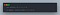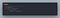# Functional programming paradigms

Functional programming paradigms: Functional programming (FP) is the most popular paradigm, because it works in the same spirit as pure functions, therefore without change of state, mutation of data and side effect.

A pure function is a function that fulfills the 2 following conditions:

• The result of the function depends only on the arguments and not on the external context
• The function has no edge effects / side effects

# ExampleJavascript program for sorting numbers in functional programming.

# Advantages and disadvantages

## Disadvantages

Although it is easy to write pure functions, it is difficult to combine them with the rest of the application as well as with the I/O. In functional programming, functions have no state, so they always create new objects to perform actions instead of modifying existing objects. For this reason, FP applications take up a lot of memory.

When a function modifies a global variable, it is no longer considered a pure function, so it may involve side effects. A side effect involving a global variable is difficult to find because it is not possible to examine parameter lists to find out which procedure/function could have modified a variable.

Functional implementation is neat, simple, contains none explicit functional loops (like `map`, `filter` or `reduce`) and is easy to modify. But it depends on several functions, including higher-order one.

Functional programming is a sub-paradigm of declarative programming. We will therefore be in a logic where we write what we want, but not necessarily how to get it.

# Imperative programing paradigms

Imperative programming paradigms: Imperative programming is a programming method describing sequences of operations in order to change the current state of the program.

An imperative code explains how to do things (it contains logic, loops, conditions, etc.).

# ExempleJavascript program for sorting numbers in imperative programming.

If we look at the program step by step:

• Create a list of results
• Review each item on the list
• Check the number, if it is even, add it to the final list.

At the end of the execution, `endArray` will be equal to `[2,4,6]`.

# Advantages and disadvantages

## Advantages

The main advantage of imperative programming is that you can control the complexity of the program. So we will try to reduce it as much as possible in order to increase its performance, as shown in the following graph.

## Disadvantages

When modifying the code, to add features or optimize it for example, the risk of errors is high.

With imperative programming, we describe what to do and how to get it. Therefore, all layers of the program must be described.

# Comparaison

The reading of this code is very easy: At first, `filter` only retrieve the parts of the form that are mandatory. In a second time, `map` allows us to get only the name of each part, and `reduce` the structure. We then obtain a result in 3 steps.

Respecting the imperative programming, we have to define and explain each step, which allows us to have control over each step. One of the solutions considered is the following:

The code is denser and it is very easy to see that it can be a source of error. At first, we loop on the list. At each iteration, we retrieve the object, and check if the object is required. If so, we get its name, and we add it as attribute to the final object.

In terms of readability, we can therefore conclude that functional programming is much easier to read and understand than imperative programming.

But what about performance? To answer this question, we are going to benchmark these two solutions thanks to the jsben.ch tool.

Before benchmarking these two solutions, we are going to verify that the functional solution is more efficient than the imperative solution with the, examples that I have used in the description of these paradigms (Follow this link to test by yourself).

The result of this test is as follows:

The functional programming is almost twice as powerful as the imperative solution.

For the case of our form, you can follow this link

After benchmarking these solutions, we obtain the following result:

It is surprising that, in this particular case, the imperative solution is more powerful than the functional solution. This is due to the fact that we master all the steps of the functionality.

However, both approaches can be mixed with the latter solution:

The list is already reduced, with an imperative condition.

When we benchmark these 3 solutions, we see that the mixed solution is the most efficient.

# Conclusion

• For small processes with a lot of data, it is preferable to use a functional solution, because it is closer to the processor, and therefore more powerful. In addition, it requires less calculation for the same result, and is therefore more ecological. This can already be noticed with the performance result of the first example.
• For more complex data processing, it is preferable to use imperative programming, because it allows you to keep control over how the code is executed, and therefore how it is optimized.
• In other cases, and especially when we are in a situation where we have several data with a complex structure, it is preferable to use a mix of these two solutions, in order to be both close to the processor, and to be able to control each program and therefore its complexity.